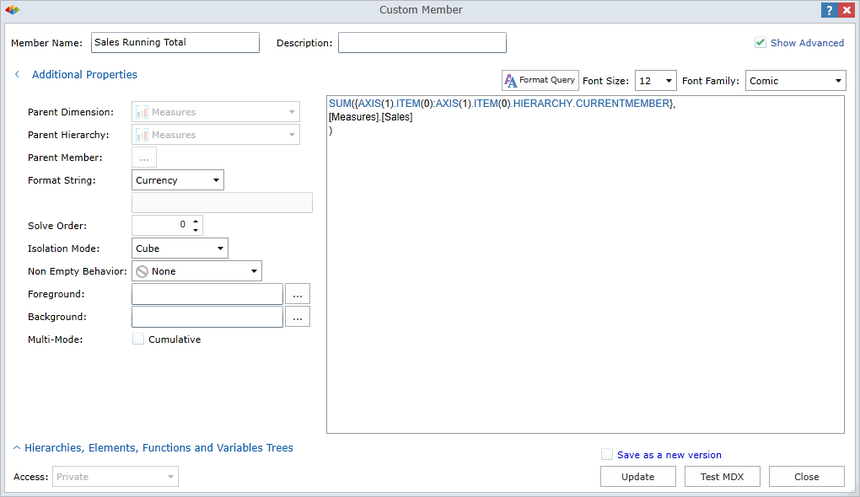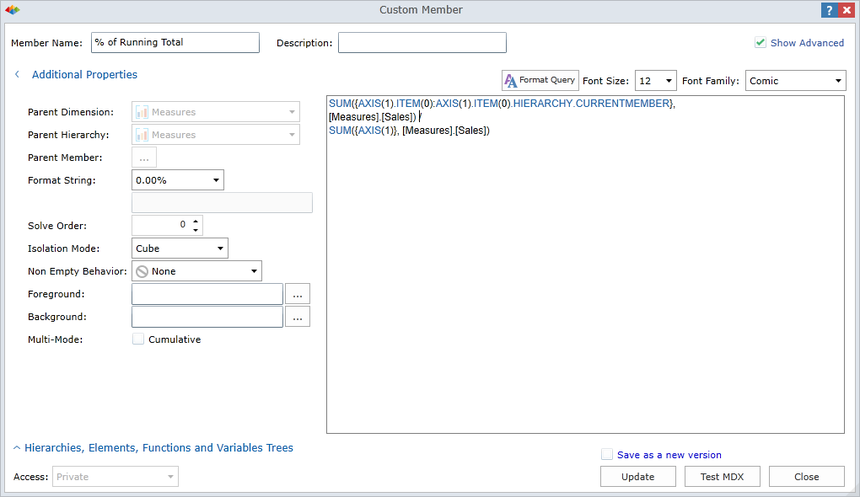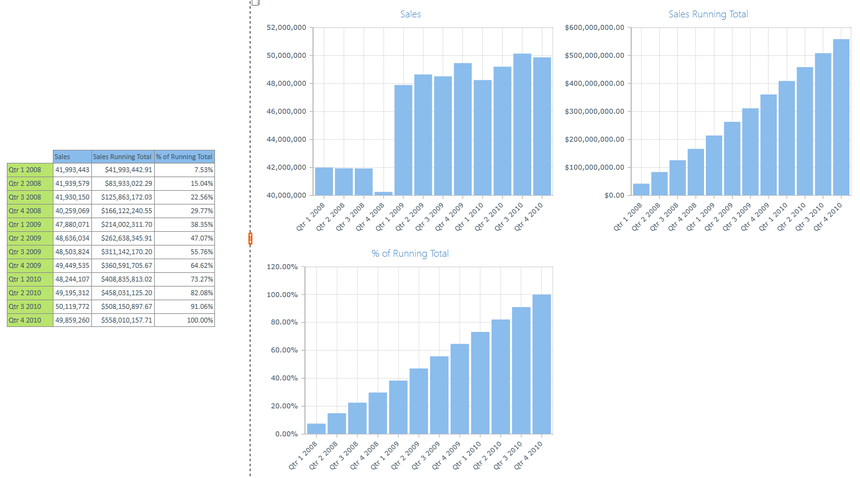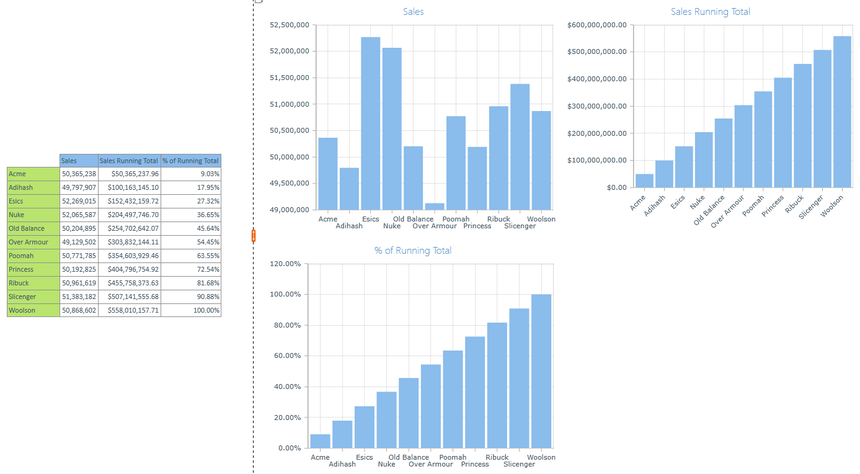0

# Axis of Awesome

by Jason Picker, Senior Sales Engineer

In your high school history class, you may have learned about the Axis of Evil, but have you heard about the Axis of Awesome?  There is a little known function in MDX called Axis that can be used for all kinds of interesting calculations.  In this blog, I will show how you can use the Axis function to create a very flexible and generic running total calculation.  This is an alternative to the approaches outlined in the blog post Creating Running Totals and Partial Sums.

## Definition

 The Axis function uses the zero-based position of an axis to return the set of tuples on an axis. For example, `Axis(0)` returns the COLUMNS axis, `Axis(1)` returns the ROWS axis, and so on.

The Axis function will return the entire set of values on a given axis.  It does not matter what dimensions or attributes are on the rows, you can calculate the running total and percentages with a simple formula.

## Running Total

SUM({AXIS(1).ITEM(0):AXIS(1).ITEM(0).HIERARCHY.CURRENTMEMBER}, [Measures].[Sales])

## Percent of Running Total

SUM({AXIS(1).ITEM(0):AXIS(1).ITEM(0).HIERARCHY.CURRENTMEMBER},

[Measures].[Sales]) / SUM({AXIS(1)}, [Measures].[Sales])

You will notice in the formula that we are only hard-coding two pieces: the Row axis and the Sales measure.  The rest is dynamically determined.  If you want to make the calculation even more generic, you could use a parameter to let the end-user pick the measure to base the calculation on instead of hard-coding it.

*NOTE:  When you use the Axis function in the Custom Member dialog, you cannot test the MDX because the Test MDX button constructs a special query that only contains a Column Axis and not a Row Axis.  Once you create the calculation, you will have to test it in a report.  Also be aware that this is not an ideal solution if you have more than one attribute hierarchy on the rows.

You will see examples of the calculations in action in figures 3 and 4 below.  I can place any dimension in the rows and the calculations will compute correctly.  This calculation is ideal if you have multiple date hierarchies in your data model.

By the way, you can use the Axis function for doing other things like computing the Average, Min, Max, or Standard Deviation values based on the set of members on the rows.  Have fun with the Axis of Awesome!Figure 1 - Running TotalFigure 2 - Percent of Running TotalFigure 3 - Calculations by TimeFigure 4 – Calculations by Manufacturer